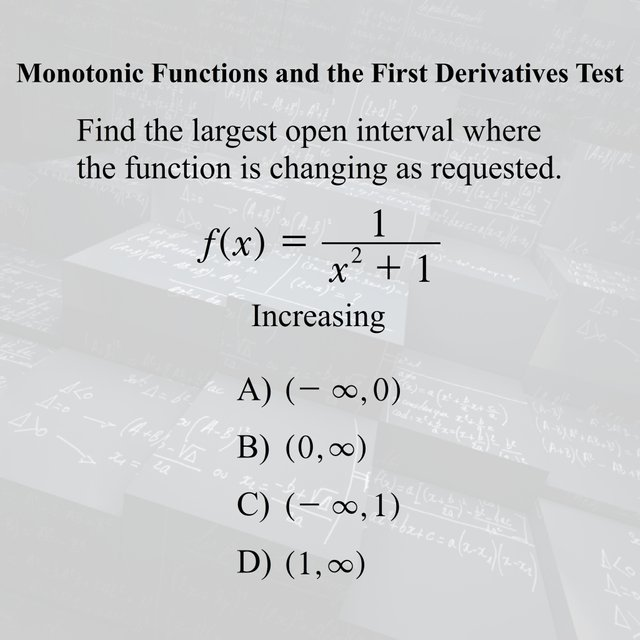# 3 Subject Math Quiz #48

in stemgeeks •  4 days ago

Try out these 3 math problems one is from Algebra I (Beginning Algebra), another one from Geometry, and the last one is from Calculus I (Differential Calculus). See if you can get all 3 correct!

Algebra IGeometryCalculus I